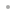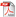MathCode F90MathCode F90FeaturesExamplesQ&ANew in Version 1.2Download ProductManualDownload InformationSheetBuy OnlineRequest a Free Trial# Examples

Simplest Example
This example shows the core functionality of MathCode F90 by compiling a trivial Mathematica function that adds two numbers. It shows how the compilation is performed and how the compiled code can be called directly from within Mathematica.

This notebook demonstrates how MathCode F90 can be used to generate Fortran code to efficiently compute a large matrix with symbolic entries. The matrix in this case is a Hessian of a given function, which is an important entity to compute efficiently. Hessians have many applications, e.g., in optimization algorithms.

The Seesaw/Pendulum Process
This notebook illustrates how to build a dynamic model of a physical system and then generate efficient code for simulation.

Find Minimum
The function FindMinimum[] finds a local minimum of a given function and a given starting value. A function cannot be translated by MathCode directly when the argument is a symbolic expression. Also, numerical algorithms for minimization are not included in the MathCode runtime library. The solution is to write a Mathematica function that replicates the FindMinimum[] operation using the compilable subset. This notebook illustrates how to do that for a function with one argument.